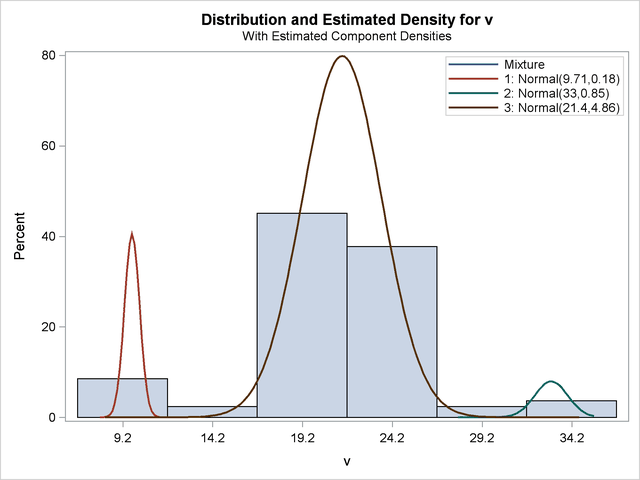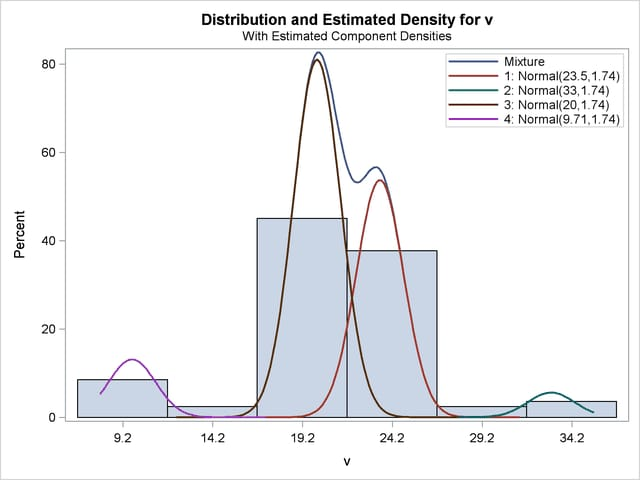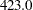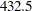Looking for Multiple Modes: Are Galaxies Clustered?

Mixture modeling is essentially a generalized form of one-dimensional cluster analysis. The following example shows how you can use PROC FMM to explore the number and nature of Gaussian clusters in univariate data.

Roeder (1990) presents data from the Corona Borealis sky survey with the velocities of 82 galaxies in a narrow slice of the sky. Cosmological theory suggests that the observed velocity of each galaxy is proportional to its distance from the observer. Thus, the presence of multiple modes in the density of these velocities could indicate a clustering of the galaxies at different distances.

The following DATA step recreates the data set in Roeder (1990). The computed variable v represents the measured velocity in thousands of kilometers per second.

```title "FMM Analysis of Galaxies Data";
data galaxies;
input velocity @@;
v = velocity / 1000;
datalines;
9172  9350  9483  9558  9775  10227 10406 16084 16170 18419
18552 18600 18927 19052 19070 19330 19343 19349 19440 19473
19529 19541 19547 19663 19846 19856 19863 19914 19918 19973
19989 20166 20175 20179 20196 20215 20221 20415 20629 20795
20821 20846 20875 20986 21137 21492 21701 21814 21921 21960
22185 22209 22242 22249 22314 22374 22495 22746 22747 22888
22914 23206 23241 23263 23484 23538 23542 23666 23706 23711
24129 24285 24289 24366 24717 24990 25633 26960 26995 32065
32789 34279
;
run;
```

Analysis of potentially multimodal data is a natural application of finite mixture models. In this case, the modeling is complicated by the question of the variance for each of the components. Using identical variances for each component could obscure underlying structure, but the additional flexibility granted by component-specific variances might introduce spurious features.

You can use PROC FMM to prepare analyses for equal and unequal variances and use one of the available fit statistics to compare the resulting models. You can use the model selection facility to explore models with varying numbers of mixture components—say, from three to seven as investigated in Roeder (1990). The following statements select the best unequal-variance model using Akaike’s information criterion (AIC), which has a built-in penalty for model complexity:

```title2 "Three to Seven Components, Unequal Variances";
ods graphics on;
ods select DensityPlot;
proc fmm data=galaxies criterion=AIC;
model v = / kmin=3 kmax=7;
ods exclude IterHistory OptInfo ComponentInfo;
run;
```

The KMIN= and KMAX= options indicate the smallest and largest number of components to consider. The ODS GRAPHICS and ODS SELECT statements request a density plot. The output for unequal variances is shown in Figure 37.14 and Figure 37.15.

Figure 37.14 Model Selection for Galaxy Data Assuming Unequal Variances
 FMM Analysis of Galaxies Data Three to Seven Components, Unequal Variances

The FMM Procedure

Model Information
Data Set WORK.GALAXIES
Response Variable v
Type of Model Homogeneous Mixture
Distribution Normal
Min Components 3
Max Components 7
Estimation Method Maximum Likelihood

Component Evaluation for Mixture Models
Model
ID
Number of -2 Log L AIC AICC BIC Pearson Max
Components Parameters
Total Eff. Total Eff.
1 3 3 8 8 406.96 422.96 424.94 442.22 82.00 0.000024
2 4 4 11 11 406.96 428.96 432.74 455.44 82.00 0.00012
3 5 5 14 14 406.96 434.96 441.23 468.66 82.00 0.000039
4 6 6 17 17 406.96 440.96 450.53 481.88 82.00 0.00012
5 7 7 20 20 406.96 446.96 460.73 495.10 82.00 0.00024

The model with 3 components (ID=1) was selected as 'best' based on the AIC statistic.

Fit Statistics
-2 Log Likelihood 407.0
AIC (smaller is better) 423.0
AICC (smaller is better) 424.9
BIC (smaller is better) 442.2
Pearson Statistic 82.0002
Effective Parameters 8
Effective Components 3

Parameter Estimates for 'Normal' Model
Component Parameter Estimate Standard Error z Value Pr > |z|
1 Intercept 9.7101 0.1597 60.80 <.0001
2 Intercept 33.0444 0.5322 62.09 <.0001
3 Intercept 21.4039 0.2597 82.41 <.0001
1 Variance 0.1785 0.09542
2 Variance 0.8496 0.6937
3 Variance 4.8567 0.8098

Parameter Estimates for Mixing Probabilities
Estimate Standard Error z Value Pr > |z|
1 Probability -2.3308 0.3959 -5.89 <.0001 0.0854
2 Probability -3.1781 0.5893 -5.39 <.0001 0.0366

Figure 37.15 Density Plot for Best (Three-Component) Model Assuming Unequal VariancesTo require that the separate components have identical variances, add the EQUATE=SCALE option in the MODEL statement:

```title2 "Three to Seven Components, Equal Variances";
ods select DensityPlot;
proc fmm data=galaxies criterion=AIC gconv=0;
model v = / kmin=3 kmax=7  equate=scale;
ods exclude IterHistory OptInfo ComponentInfo;
run;
```

The GCONV= convergence criterion is turned off in this PROC FMM run to avoid the early stoppage of the iterations when the relative gradient changes little between iterations. Turning the criterion off usually ensures that convergence is achieved with a small absolute gradient of the objective function.

The output for equal variances is shown in Figure 37.16 and Figure 37.17.

Figure 37.16 Model Selection for Galaxy Data Assuming Equal Variances
 FMM Analysis of Galaxies Data Three to Seven Components, Equal Variances

The FMM Procedure

Model Information
Data Set WORK.GALAXIES
Response Variable v
Type of Model Homogeneous Mixture
Distribution Normal
Min Components 3
Max Components 7
Estimation Method Maximum Likelihood

Component Evaluation for Mixture Models
Model
ID
Number of -2 Log L AIC AICC BIC Pearson Max
Components Parameters
Total Eff. Total Eff.
1 3 3 6 6 478.74 490.74 491.86 505.18 82.00 1.197E-6
2 4 4 8 8 416.49 432.49 434.47 451.75 82.00 6.967E-7
3 5 5 10 10 416.49 436.49 439.59 460.56 82.00 4.31E-6
4 6 6 12 12 416.49 440.49 445.02 469.37 82.00 3.03E-6
5 7 7 14 14 416.49 444.49 450.76 478.19 82.00 4.896E-6

The model with 4 components (ID=2) was selected as 'best' based on the AIC statistic.

Fit Statistics
-2 Log Likelihood 416.5
AIC (smaller is better) 432.5
AICC (smaller is better) 434.5
BIC (smaller is better) 451.7
Pearson Statistic 82.0000
Effective Parameters 8
Effective Components 4

Parameter Estimates for 'Normal' Model
Component Parameter Estimate Standard Error z Value Pr > |z|
1 Intercept 23.5058 0.3460 67.93 <.0001
2 Intercept 33.0440 0.7610 43.42 <.0001
3 Intercept 20.0086 0.3029 66.06 <.0001
4 Intercept 9.7103 0.4981 19.50 <.0001
1 Variance 1.7354 0.3905
2 Variance 1.7354 0.3905
3 Variance 1.7354 0.3905
4 Variance 1.7354 0.3905

Parameter Estimates for Mixing ProbabilitiesNot surprisingly, the two variance specifications produce different optimal models. The unequal variance specification favors a three-component model while the equal variance specification favors a four-component model. Comparison of the AIC fit statistics,and, indicates that the three-component, unequal variance model provides the best overall fit.